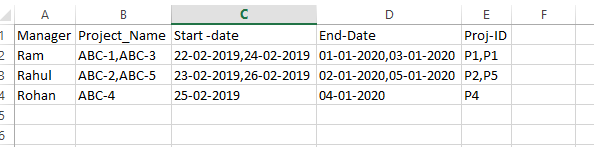# Can someone assist me how to extract the following data from excel

I have a excel sheet which has few columns like Emp name, Proj ID, Manager name, Project name, Start date, End date
One emp name is associated with more than one project and also one manager would be associated with multiple projects.
How do i extract for each emp all his unique Proj IDs, project names, start date and end date
Also for each manger : his unique Proj IDs, Project Names, start date and end date

Excel_Data.xlsx (9.7 KB)

in general we can do it with grouping the data:

About the output. It would be helpfully if you can specified it more in detail e.g. for each manager a worksheet…

Thanks for the responseIn the above image, For Eg: Kevin name is found on 2 rows, So i need to extract
Project Names(ABC-1, ABC-2) , Project IDS(P1 and P1), Start dates and end dates for Kevin

Hi @shilpa_p ,

Is this the expected output?If so then give this workflow a try →

Employee →

``````dt_sampleData.AsEnumerable().GroupBy(Function(g) g("Emp_name").ToString).
so.Key,String.Join(",",so.Select(Function(s) s("Project_Name").ToString)),
String.Join(",",so.Select(Function(s) Convert.ToDateTime(s("Start -date").ToString).ToString("dd-MM-yyyy"))),
String.Join(",",so.Select(Function(s) Convert.ToDateTime(s("End-Date").ToString).ToString("dd-MM-yyyy"))),
String.Join(",",so.Select(Function(s) s("Proj-ID").ToString))},False)).CopyToDataTable()
``````

Manager →

``````dt_sampleData.AsEnumerable().GroupBy(Function(g) g("Manager").ToString).
so.Key,String.Join(",",so.Select(Function(s) s("Project_Name").ToString)),
String.Join(",",so.Select(Function(s) Convert.ToDateTime(s("Start -date").ToString).ToString("dd-MM-yyyy"))),
String.Join(",",so.Select(Function(s) Convert.ToDateTime(s("End-Date").ToString).ToString("dd-MM-yyyy"))),
String.Join(",",so.Select(Function(s) s("Proj-ID").ToString))},False)).CopyToDataTable()
``````

ExtractEmpAndManagerDetails.xaml (9.1 KB)

Kind Regards,
Ashwin A.K

it is a group by case and can be implemented as described in the link shared above

With your input: Project Names(ABC-1, ABC-2) , Project IDS(P1 and P1) we do have a requirement of concatening the group member values (PName, PIDs)

about the start date there is no requirment given. it could be the case to use the lowest startdate of the kevin projects and the latest End date for the kevon projects in the case of Kevin. But as it is an assumption we would prefer to have all definitons together before we start

Yes i was looking for a similar kind.
I will try with this
Thanks a lotThis works perfect for my input file which is on similar basis. Thanks a lot

Is there any way that instead of joining by ", " can i add it to the next line

You mean like this?If so, then simply edit the delimiter to vbNewline →

Kind Regards,
Ashwin A.K

1 Like